## Nonlinear isotropic material for moisture transport - NlIsoMoisture

This is a more general model for nonlinear moisture transport in isotropic porous materials, based on a nonlinear sorption isotherm (relation between the pore relative humidity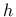and the water content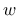) and on a humidity-dependent moisture permeability. The governing differential equation reads

 (237)

where [kg/m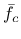] is the humidity-dependent moisture capacity (derivative of the moisture content with respect to the relative humidity), and [kg/m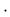s] is the moisture permeability.

So far, six different functions for the sorption isotherm have been implemented (in fact, what matters for the model is not the isotherm itself but its derivative--the moisture capacity):

1. Linear isotherm ( ) is characterized only by its slope given by parameter .

2. Piecewise linear isotherm ( ) is defined by two arrays with the values of pore relative humidity and the corresponding values of moisture content . The arrays must be of the same size.

3. Ricken isotherm  ( ), which is widely used for sorption of porous building materials. It is expressed by the equation

 (238)

where [kg/m] is the water content at and [m/kg] is an approximation coefficient. In the input record, only must be specified ( is not needed). Note that for this isotherm gives an infinite moisture content.

4. Isotherm proposed by Kuenzel  ( ) in the form

 (239)

where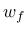[kg/m] is the moisture content at free saturation and is a dimensionless approximation factor greater than 1.

5. Isotherm proposed by Hansen  ( ) in the form

 (240)

characterizes the amount of adsorbed water by the moisture ratio [kg/kg]. To obtain the moisture content, it is necessary to multiply the moisture ratio by the density of the solid phase. In (240), is the maximum hygroscopically bound water by adsorption, and and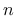are constants obtained by fitting of experimental data.

6. The BSB isotherm  ( ) is an improved version of the famous BET isotherm. It is expressed in terms of the moisture ratio

 (241)

where is the monolayer capacity, and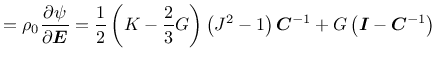depends on the absolute temperature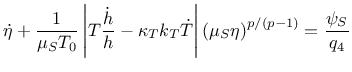and on the difference between the heat of adsorption and condensation. Empirical formulae for estimation of the parameters can be found in . Note that these formulae hold quite accurately for cement paste only; a reduction of the moisture ratio is necessary if the isotherm should be applied for concrete.

The present implementation covers three functions for moisture permeability:

1. Piecewise linear permeability ( ) is defined by two arrays with the values of pore relative humidity and the corresponding values of moisture content . The arrays must be of the same size.

2. The Bazant-Najjar permeability function ( ) is given by the same formula (236) as the diffusivity in Section 2.3. All parameters have a similar meaning as in (236) but is now the moisture permeability at full saturation [kg/ms].

3. Permeability function proposed by Xi et al.  ( ) reads

 (242)

where , and are parameters that can be evaluated using empirical mixture-based formulae presented in . However, if those formulae are used outside the range of water-cement ratios for which they were calibrated, the permeability may become negative. Also the physical units are unclear.

Note that the Bajant-Najjar model from Section 2.3 can be obtained as a special case of the present model if is set to 1 and is set to 0. The ratio then corresponds to the diffusivity parameterfrom Eq. (235).

The model parameters are summarized in Tab. 55.

Table 55: Nonlinear isotropic material for moisture transport - summary.
 Description Nonlinear isotropic material for moisture transport Record Format NlIsoMoistureMat num(in) # d(rn) # isothermType(in) # permeabilityType(in) # [ rhodry(rn) #] [ capa(rn) #] [ iso_h(ra) #] [ iso_w(h)(ra) #] [ dd(rn) #] [ wf(rn) #] [ b(rn) #] [ uh(rn) #] [ A(rn) #] [ nn(rn) #] [ c(rn) #] [ k(rn) #] [ Vm(rn) #] [ perm_h(ra) #] [ perm_c(h)(ra) #] [ c1(rn) #] [ n(rn) #] [ alpha0(rn) #] [ hc(rn) #] [ alphah(rn) #] [ betah(rn) #] [ gammah(rn) #] Parameters - num material model number - d material density - isothermType isotherm function as listed above (0, 1, ...5) - permeabilityType moisture permeability function as listed above (0, 1, 2) - rhodry [kg/m] density of dry material (for and 5) - capa [kg/m] moisture capacity (for ) - iso_h [-] humidity array (for ) - iso_w(h) [kg/m] moisture content array (for ) - dd [-] parameter (for ) - wf [kg/m] is the moisture content at free saturation (for ) - b [-] parameter (for ) - uh [kg/kg] maximum hygroscopically bound water by adsorption (for ) - A [-] parameter (for ) - n [-] parameter (for ) - Vm (for ) - k (for ) - C (for ) - perm_h [-] humidity array (for ) - perm_c(h) [kg ms] moisture permeability array (for ) - c1 [kg ms] moisture permeability at full saturation (for ) - n [-] exponent (for ) - alpha0 [-] ratio between minimum and maximum diffusivity (for ) - hc [-] relative humidity at which the diffusivity is exactly between its minimum and maximum value (for ) - alphah [kg ms] (for ) - betah [kg ms] (for ) - gammah [-] (for ) Supported modes _2dHeat

Borek Patzak
2018-01-02